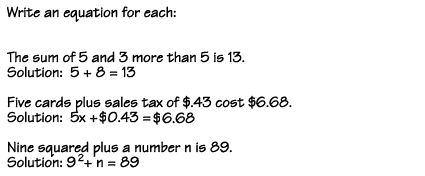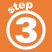Home    |    Teacher    |    Parents    |    Glossary    |    About UsThere are several tips to help you keep track of algebraic expressions.

What was that variable?
It's a good idea to write down what your variables mean. For example, write this equation: Today a boy is twice as old as he was 4 years ago.

Let b = the boy's age today

b = 2 (b - 4 years)
b = 2b - 8 years
b = 8 years

The boy is 8 years old today. A common mistake would be to get confused whether the answer was the age today or 4 years ago. Of course, this is a simple example, but as your equations get more complicated, it is very important to keep track of what your variables represent.

Units
Remember to include units. If you have a real-world problem that uses units, keeping the units in the equation will help you figure it out.

For example, write this expression - The cost was \$2.00 less than half of \$5.00

Let x = the cost

x = 1/2 (\$5.00) - \$2.00
x = \$2.50 - \$2.00
x = \$0.50 or fifty cents

Without the units, It would be easy to lose track of what the answer of .5 meant. Again, this is a simple example, but as things get more complicated, it's important to keep units straight.

 Homework Help | Algebra | The Language of AlgebraEmail this page to a friendSearch·  Definitions·  Order of Operations·  Writing equations·  Writing inequalitiesFirst Glance In Depth Examples WorkoutWriting equations